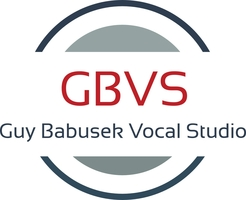### 4 Pack -- 30-Minute Lessons

\$280.00
Buy a package of 4 lessons and receive a \$5 per lesson discount off the single lesson price.

This package is a \$20 total savings.

### 8 Pack -- 30-Minute Lessons

\$540.00
Buy a package of 8 lessons and receive a \$7.50 discount per lesson off the single lesson price.

This package is a \$60 total savings.

### 12 Pack -- 30-Minute Lessons

\$780.00
Buy a package of 12 lessons and receive over \$10 per lesson off the single lesson price.

This package is a \$120 total savings.

### 16 Pack -- 30 Minute Lessons

\$960.00
Buy a package of 16 lessons and receive a \$15 discount per lesson off the single lesson price.

This package is a \$240 total savings.

### 32 Pack Deep Discount HALF HOUR

\$1,700.00
Series of 32 Half-Hour Lessons at the largest discount I offer.

### 4 Pack -- One-Hour Lessons

\$540.00
Buy a package of 4 lessons, and receive an additional \$5 discount per lesson off the single lesson price!

Hour lessons are already discounted \$10 per lesson from the 1/2 hour price structure.

This package is a \$60 total savings.

### 8 Pack -- One-Hour Lessons

\$1,060.00

Hour lessons are already discounted \$10 per lesson from the 1/2 hour price structure.

This package is a \$140 total savings.

### 12 Pack -- One-Hour Lessons

\$1,560.00
Buy a package of 12 lessons, and receive an additional \$10 discount per lesson off the single lesson price.

Hour lessons are already discounted \$10 per lesson from the 1/2 hour price structure.

This package is a \$240 total savings

### 16 Pack -- One Hour Lessons

\$2,000.00
Buy a package of 16 lessons, and receive an additional \$15 discount per lesson off the single lesson price

Hour lessons are already discounted by \$10 per lesson off the 1/2 hour pricing structure.

This package is a \$400 total savings.

### 32 Pack Deep Discount HOUR

\$3,700.00
Series of 32 One Hour Lessons at the largest discount I offer.

### 64 Pack Deep Discount HOUR

\$5,760.00
This is the lowest price I currently offer. \$90 per hour.